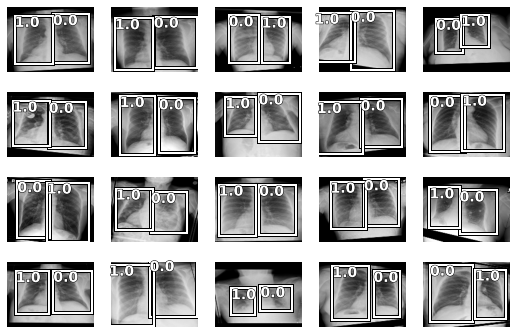# Labels of bounding boxes shifted as show_batch() result

Hello everyone,

I’m developing a project about the lecture of X-rays images of COVID-19 patients.
It’s my first time developing a Deep Learning project.

I’m adapting the original code that the professors supplied to me.
The first goal and the one I’m trying to reach now is to detect and classify properly the lungs of the images from the dataset.

I’ve created the bounding boxes of the lungs of all the images by using the LabelImg software (https://github.com/tzutalin/labelImg). For each image, a .txt in YOLO format is created with 2 lines such as the following:

0 0.290076 0.484733 0.423664 0.812977
1 0.762405 0.499046 0.417939 0.822519

The first line is associated to the bounding box of the left lung and the second line is for the bbox of the right lung.
On each line, the first value is the id of the class of the bounding box and the other values are it’s data (center_x, center_y, width and height relative to the image).

I’ve been able to create a DataBlock() with no errors, but when I run the show_batch() function some images have the labels shifted as you can see in the next attached image. The left lungs must have 0.0 as label, but in some images the label is 1.0.I don’t know why in some images the labels are associated correctly to the bounding boxes and in some others they aren’t.
First I reviewed how I labeled the images on the LabelImg to check if some .txt had the classes ids shifted but it wasn’t the case.
Then I reviewed how I stored the labels on the get_labels() function [See code below] and they’re stored with the correct order. I iterate each .txt and get the class ids.
I also tried to debug the Python notebook with the debugger of VS Code to try to find how the labels where stored in the DataBlock object but I didn’t find any field related to the labels.
Finally, I searched on the forums of Fastai to find some related topic but I found none.

I would gradly appreciate if anyone can give me any clue of how to solve the problem related before.
At the end of this message you can find the key parts of my code.

Thank you.

Marc

``````def get_bboxes(f):

img = PILImage.create(path+f)

# Get the annotations of the bounding boxes of the lungs of the rx image with filename "f"
fullAnnot = np.genfromtxt(img2txt_name(Path(f)))

bboxes = np.zeros((2,4))

for i in range(len(fullAnnot)):
cx = int(fullAnnot[i]*img.size)
cy = int(fullAnnot[i]*img.size)

w = int(fullAnnot[i]*img.size)
h = int(fullAnnot[i]*img.size)

# careful! fastaiv2 change x coordinate first!
#minx miny maxX maxY
bbox= np.zeros(4)
bbox = float(cx-w/2.0)#/img.size#*img_size
bbox = float(cy-h/2.0)#/img.size#*img_size
bbox = float(cx+w/2.0)#/img.size#*img_size
bbox = float(cy+h/2.0)#/img.size#*img_size

bboxes[i] = bbox

return bboxes

def get_labels(f):
fullAnnot = np.genfromtxt(img2txt_name(Path(f)))
labels = fullAnnot[:,0]
return labels

get_y = [lambda o: get_bboxes(o.name), lambda o: get_labels(o.name)] # lambda o: means for each file on the dataset, o.name = filename
``````
``````tfms = [*aug_transforms(max_zoom=1, max_warp=0.05, max_rotate=0.05, max_lighting=0.2),Normalize.from_stats(*imagenet_stats)]

# * before aug_transforms is used to unpack every element of the aug_transforms list, and then store them in a list called tfms

tfms = []

data = DataBlock(

blocks=(ImageBlock, BBoxBlock,BBoxLblBlock), # ImageBlock means type of inputs are images; BBoxBlock & BBoxLblBlock = type of targets are BBoxes & their labels

get_items=get_image_files,

n_inp=1, # number of inputs; it's 1 because the only inputs are the rx images (ImageBlock)

get_y=get_y, # get_y = targets [bboxes, labels]; get_x = inputs

splitter = RandomSplitter (0.1), # split training/validation; parameter 0.1 means there will be 10% of validation images

batch_tfms= [*aug_transforms(size=(120,160)), Normalize.from_stats(*imagenet_stats)]

)
``````
``````path_dl = Path(path)
Path.BASE_PATH = path_dl
path_dl.ls().sorted()
``````
``````dls = data.dataloaders(path_dl, path=path_dl, bs = 64)
dls.show_batch(max_n=20, figsize=(9,6))
``````
1 Like

Thank you for all the detail. I think what is happening is that your images are flipping due to your augmentations that are being applied. If that isn’t an appropriate augmentation for your data, you can turn that option off from `aug_transforms`. Data augmentation in computer vision | fastai

`do_flip=True` by default, but you probably want to set that to false in this use-case

1 Like

Thank you so much.

I set the argument of aug_transforms() do_flip=False and now every image of the batch has the bbox left with the label 0.0 and the bbox right with the label 1.0.